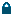Log In
 EN CHOOSE LANGUAGE ENGLISH

# Signalator Results Notions

Signalator website includes hundreds of pages. Most of these pages are results-related. To give a full view of our forex signals results, we use quite a big number of various terms, notions and definitions. Most likely, you are more than familiar with the most of these values. However, if you are not sure about any notion, click on a question (?) sign next to a notion or refer to this page to check what a notion means. Further, you will find the list of the most used notions and definitions. The following list is updated on a permanent basis.

Total Profit - May also be referred as a Net Profit, Total Net Profit. The sum of all trades made. Total Profit = Profitable Trades Results - Loss Trades Results. Higher values are better.

Winning Ratio (Win Ratio) - The ratio between profitable and all trades. Win Ratio = Profitable Trades / All Trades. Higher values are better.

Average profit a month - Indicates an average monthly profit. Average Profit a Month = Total Profit / Total Months. Higher values are better.

Weighted average profit a month - Weighted average value with the most recent results having the highest weight. The default type of average used by Signalaor. Most recent results matter more than old results.

Risk-reward ratio - The ratio between an expected level of TP and an expected level of SL. Risk Reward Ratio = TP Level / SL Level. Higher values are better.

Months traded - May also be referred as a Total Trading Period, Total Months. A total number of months traded. Months Traded = Total Number of Months (Date End - Date Start) Basically the longer a signal is traded the more stable it is.

Recommended deposit info - A recommended level of deposit to allocate for 0.1 lot trading. If you trade 0.1 lot, on average 1 pip = \$1. Detailed information about the recommended deposits, you may find here.

Average number of trades a month - An average number of trades made during one calendar month. Average Number of Trades a Month = Total Trades / Total Months .

Net profit ratio (Efficiency Ratio) - Represents a net profit to total gains ratio. Net Ratio = Net Profit / Total Gains Higher values are better.

RoMaD (Return over maximum drawdown) - Represents a ratio between relative net income (net income in percentage) to the maximum drawdown level.
RoMaD = Net Profit in % / Max Drawdown % *-1
Higher values are better.

TP total / ratio - A number of trades closed by TP (take profit) and the ratio to all trades. TP Ratio = TP Trades Number / All Trades Higher values are better

Pairs included - A number of currency pairs included within a trading signals set. Valid for a signals set only. Discover all Signalator signals sets.

Diversification factor - A ratio between a cumulative drawdown levels and a level of drawdown received if traded with all included pairs. Diversification Factor = Sum of Drawdown Values of Each Pair / Drawdown of a Signals SetHigher values are better. Valid for a signals set only.

Monthly win ratio - A ratio between profitable months and all traded months . Monthly Win = Profitable Months / Total Months Higher values are better.

Profit received - A total amount of gains (profitable trades results). Total Gains = Sum of All Profitable Trades Results

Profitable months - A number of profitable months. A profitable month = a month closed with a positive result.

Best month - May also be referred as a most profitable month. The most profitable month within all traded month. A month with the highest profit.

Drawdown - Maximum reduction / decrease of an equity. The maximum decline of a capital from a maximum to a subsequent minimum. The lower, the better.

Total loss - May also be referred as a Total Loss Received. A total amount of losses. Total Losses = Sum of All Loss Trades Results